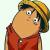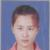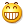# 关于运算符重载的几点

Linux小菜 发布于 2014/04/28 22:52

## 1.运算符重载

C++中，除了可以对函数重载外，还可以对运算符重载，通过创建运算符函数 operator() 来实现，一般运算符重载作用在类上 。函数operator() 既可以是它将要操作的类的成员，也可以不是类成员，如果不是类成员，必须是类的友元函数。

class Test

{

private:

int m_a;

public:

Test(int a):m_a(a){}

};

int main()

{

Test t1(1);

Test t2(2);

t2 + t1;    //报错,需要重载运算符"+"

}

C++规定，运算符中， 参数说明都是内部类型时，不能重载。例如不允许声明：

int operator(int ,int) ;   //,因为这种重载没意义

C++还规定了“．、:: 、．->*?：” 这五个运算符不能重载，也不能创造新运算符。

#include <iostream>

using namespace std;

class Testpm

{

public:

void m_func1()

{

cout << "m_func1\n";

}

int m_num;

};

//定义指向类成员函数的指针

void (Testpm::*pmfn)() = &Testpm::m_func1;

//定义指向类成员数据的指针

int Testpm::*pmd = &Testpm::m_num;

int main() {

Testpm ATestpm;               //创建一个类对象

Testpm *pTestpm = new Testpm; //创建类对象的指针

//对象 用指向类成员的指针 去访问对象成员

(ATestpm.*pmfn)();

ATestpm.*pmd = 1;

cout  << ATestpm.*pmd << endl;

//对象指针 用指向类成员的指针 去访问对象成员

(pTestpm->*pmfn)();

pTestpm->*pmd = 2;

cout<< pTestpm->*pmd << endl;

delete pTestpm;

return 0;

}

1，成员运算符重载。

2，友元运算符函数(非友元的普通函数不能访问类的私有成员)

1.1 成员运算符函数

C++规定：=()[]->这四种运算符必须为成员形式

class X

{

//....

Type operator @(参数表);

//....

};

Type  X :: operator @(参数表)

{

//.....

1，单目成员运算符重载（参数表为空）。

2，双目成员运算符重载（参数表中有一个参数）.

1.1.1单目成员运算符重载

@obj ;                 //隐式调用

Obj.operator @() ;        //显式调用, operator @ 是一个函数名字

#include <iostream>

using namespace std;

//注：重载单目运算符，默认前缀

class Test

{

public:

Test(int a,int b):m_a(a),m_b(b) //构造函数的初始化列表

{}

Test & operator ++()            //类成员重载单目运算符"++"

{

m_a++;

m_b++;

return *this;               //返回当前对象的引用

}

void prt()

{

cout<<m_a<<" "<<m_b<<endl;

}

private:

int m_a;

int m_b;

};

int main()

{

Test t(1,2);

t.prt();          //输出结果 1 2

++t;              //隐式调用

// t++;           //error, 因为单目运算符重载，默认是前缀的

//t.operator ++();  //显示调用

t.prt();          //输出结果 2 3

++++t;            //隐式调用

//t.operator ++().operator ++(); //显示调用

t.prt();          //输出结果 4 5  ,如果将上面重载函数的引用&去掉则结果3 4

return 0;

}

/*

*/

1.1.2 双目成员运算符重载

class X

{

//....

int operator +(X  a);

};

一般而言，双目成员运算符函数重载时，可以用以下两种方法来使用：

aa @ bb  ;               //隐式调用

aa.operator @ (bb) ;        //显式调用, operator @ 是一个函数名字

#include <iostream>

using namespace std;

class Test

{

public:

Test(){}    //默认的无参构造函数

Test(int a,int b):m_a(a),m_b(b) //有参构造函数的初始化列表

{}

Test operator +(const Test &obj)      //类成员重载双目运算符"+"

{

Test temp;

temp.m_a = this->m_a + obj.m_a;

temp.m_b = this->m_b + obj.m_b;

return temp;               //返回临时对象

}

void show()

{

cout<<m_a<<" "<<m_b<<endl;

}

private:

int m_a;

int m_b;

};

int main()

{

Test t1(1,2);

Test t2(2,3);

Test t3 = t1 + t2;             //显示调用

Test t4 = t1.operator +(t2);   //隐式调用

Test t5 = t1 + t2 + t3;        //重载“+”运算符的函数返回类对象，这里才能连续使用，否则不行

t3.show();

t4.show();

t5.show();

return 0;

}

/*

*/

2.1 友元运算符函数

friend  type operator @( 参数表 );

1.单目友元运算符重载函数

2.双目友元运算符重载函数

2.1.1 单目友元运算符重载函数

@ aa ;            //隐式调用

operator @ (aa ) ;  //显示调用，operator @ 是一个函数名字

#include <iostream>

using namespace std;

class Test

{

public:

Test(){}    //默认的无参构造函数

Test(int a):m_a(a){} //有参构造函数的初始化列表

friend Test & operator ++(Test &obj)//友元重载单目运算符"+",参数一定要引用

{

++obj.m_a;

return obj;

}

void show()

{

cout<<m_a<<endl;

}

private:

int m_a;

};

int main()

{

Test t1(1);

++t1;            //隐式调用

//  t1++ ;       //error, 因为单目运算符重载，默认前缀

t1.show();

Test t2(1);

operator ++(t2); //显示调用, operator ++ 是函数名

t2.show();

Test t3(1);

++++t3;

t3.show();

return 0;

}

2.1.2 双目友元运算符重载函数

aa @ bb ;            //隐式调用

operator @ (aa , bb) ;  //显示调用，operator @ 是一个函数名字

#include <iostream>

using namespace std;

class Test

{

public:

Test(){}

Test(int a):m_a(a){}

friend Test operator +(const Test &obj1,const Test &obj2); //友元重载"+"

void show()

{

cout<<m_a<<endl;

}

private:

int m_a;

};

/*参数一定要加const修饰，原因：双目运算符操作数不应该被改变，所以编译器内部有校验，判断在函数内部能不能去修改外部操作数的值。如果能改，那么

Test operator +(const Test &obj1,const Test &obj2)

{

Test temp;

temp.m_a = obj1.m_a + obj2.m_a;

return temp;

}

int main()

{

Test t1(1);

Test t2(2);

Test t3 = t1 + t2;           //隐式调用

Test t4 = operator +(t1,t2); //显示调用，operator + 是函数名

Test t5 = t1 + t2 + t3;

t3.show();

t4.show();

t5.show();

}

:VC++6.0 如果没打相应的补丁，是不支持友元双目重载的，如上面代码就在VS6.0下 编译不成功.

3. 成员运算符重载和友元运算符重载的比较

1）对单目运算符而言，成员运算符函数不带参数，而友元带一个参数

2）对双目运算符而言，成员运算符函数带一个参数，而友元带二个参数。

分析上面描述，会发现友元运算符重载函数会比成员的多一个参数，原因： 成员函数有一个隐藏的this指针 指向调用对象.

Test operator +(int a)

{

Test temp;

temp.m_a =  m_a + a;

return temp;

}

Test t1(1);

Test t2(2);

Test t3 = t1 + 2;    //OK

//Test t4 = 2 + t1;  //error, 这样写相当于: 2.operator(t1)

#include <iostream>

using namespace std;

class Test

{

public:

Test(){}

Test(int a):m_a(a){}

friend Test operator +(const Test &obj,int a)

{

Test temp;

temp.m_a =  obj.m_a + a;

return temp;

}

friend Test operator +(int a,const Test &obj)

{

Test temp;

temp.m_a =  obj.m_a + a;

return temp;

}

void show()

{

cout<<m_a<<endl;

}

private:

int m_a;

};

int main()

{

Test t1(1);

Test t2(2);

Test t3 = t1 + 2;    //OK

Test t4 = 2 + t1;    //OK

t3.show();

t4.show();

return 0;

}

4. 单目运算符的后缀形式

#include <iostream>

using namespace std;

class Test

{

public:

Test(){}

Test(int a):m_a(a){}

Test& operator ++();

Test& operator ++(int);

friend Test& operator --(Test &obj);

friend Test& operator --(Test &obj,int);

void show()

{

cout<<m_a<<endl;

}

private:

int m_a;

};

Test& Test::operator ++()

{

cout<<"成员调用前缀++"<<endl;

m_a++;

return *this;

}

Test& Test::operator ++(int)

{

cout<<"成员调用后缀++"<<endl;

m_a++;

return *this;

}

Test& operator --(Test &obj)

{

cout<<"友元调用前缀--"<<endl;

obj.m_a--;

return obj;

}

Test& operator --(Test &obj,int)

{

cout<<"友元调用后缀--"<<endl;

obj.m_a--;

return obj;

}

int main()

{

Test t1(1);

++t1;

t1++;

t1.operator ++();  //等价于 ++t1;

t1.operator ++(0); //等价于 t1++;

Test t2(1);

--t2;

t2--;

operator --(t2);  //等价于 --t2;

operator --(t2,0);//等价于 t2--;

return 0;

}

5. 赋值运算符 =

Test &  operator =(const Test & obj)

{

//每个成员的依次简单赋值

}

Test  t1 = t2;   //在创建对象时，这里调用构造函数不是调用赋值运算符函数

Test t1,t2;

t1 = t2;      //这里调用的是赋值运算符函数

#include <iostream>

using namespace std;

#include <string.h>

class MyString

{

public:

MyString()

{

m_str = new char;

*m_str = '\0';

}

MyString(const char * str)

{

m_str = new char[strlen(str)+1];

strcpy(m_str,str);

}

MyString(const MyString &obj)  //

{

m_str = new char[strlen(obj.m_str)+1];

strcpy(m_str,obj.m_str);

}

~MyString()

{

delete []m_str;

}

MyString & operator =(const MyString & obj) //赋值运算符重载

{

delete []m_str;

m_str = new char[strlen(obj.m_str)+1];

strcpy(m_str,obj.m_str);

return *this;

}

void show()

{

cout<<m_str<<endl;

}

private:

char *m_str;

};

int main()

{

MyString s1("hello");

MyString s2 = s1;

s2.show();

MyString s3;

s3="world";  /*注意：这个地方，产生了隐式转换，char * 转换为MyString对象。实际情况是，创建了临时对象MyString("world"),能够产生这种隐士转换的前提是提供了相应的构造函数，否则，不能隐士转换*/

s3.show();

return 0;

}

6. 运算符（）和[ ] 的重载

6.1 运算符（）的重载

()运算符是一个双目运算符

（）运算符的调用形式：

obj(参数表)           //隐式调用

obj.operator ()(参数表)  //显示调用

#include <iostream>

using namespace std;

class Test

{

public:

Test(){};

Test(int a,int b):m_a(a),m_b(b){}

Test& operator ()(int a,int b)  //重载运算符()

{

m_a = a;

m_b = b;

return *this;

}

void show()

{

cout<<m_a<<"  "<<m_b<<endl;

}

private:

int m_a;

int m_b;

};

int main()

{

Test t(1,2);

t.show();

t(3,4);     //隐式调用

t.show();

t.operator ()(7,8);  //显示调用，等价于 t(7,8)

t.show();

return 0;

}

6.2 运算符[ ]的重载

[ ]运算符是一个双目运算符

[ ] 运算符的调用形式：

obj[形参]             //隐式调用

obj.operator [](形参)  //显示调用

注意：形参只能有一个，和我们以前的数组下标一样的使用

#include <iostream>

using namespace std;

#include <string.h>

class MyString

{

public:

MyString()

{

m_str = new char;

*m_str = '\0';

}

MyString(const char * str)

{

m_str = new char[strlen(str)+1];

strcpy(m_str,str);

}

MyString(const MyString &obj)

{

m_str = new char[strlen(obj.m_str)+1];

strcpy(m_str,obj.m_str);

}

~MyString()

{

delete []m_str;

}

char & operator [](int n)  //要返回引用，因为只有这样才能作为=的左值

{

return m_str[n];

}

void show()

{

cout<<m_str<<endl;

}

0